﻿ 微型燃烧器扩展角对氢气/空气预混燃烧特性影响的模拟研究 Simulation Study on the Influence of Micro Burner Expansion Angle on Hydrogen/Air Premixed Combustion Characteristics

Modeling and Simulation
Vol. 10  No. 01 ( 2021 ), Article ID: 40374 , 14 pages
10.12677/MOS.2021.101010

1上海理工大学能源与动力工程学院，上海

2上海船用柴油机研究所，上海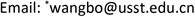Simulation Study on the Influence of Micro Burner Expansion Angle on Hydrogen/Air Premixed Combustion Characteristics

Zhuo Wang1, Zhipeng Yu2, Peng Ji2, Bo Wang1*, Menghui Liu1, Chengxi Sun1

1School of Energy and Power Engineering, University of Shanghai for Science and Technology, Shanghai

2Shanghai Marine Diesel Engine Research Institute, ShanghaiReceived: Nov. 20th, 2020; accepted: Feb. 2nd, 2021; published: Feb. 10th, 2021ABSTRACT

Based on the established three-dimensional model of the microburner, the premixed combustion process of hydrogen/air in a 100 mm long microburner with expansion angles of 0˚, 0.36˚ and 0.72˚ was simulated with 10% hydrogen volume fraction. It is found that for square micro-channel burners, with the same burner length, outer wall length and inlet size, the larger the burner expansion angle, the larger the speed limit range. When the inlet velocity is low, the larger the spreading angle is, the lower the flame propagation velocity is, and the higher the maximum combustion temperature is; when the inlet velocity is high and the gas is fully burned, the expansion angle has little effect on the maximum combustion temperature.

Keywords:Micro Burner, Expansion Angle, Inlet Velocity, Maximum Combustion Temperature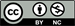1. 引言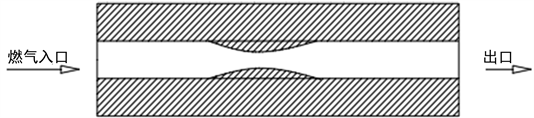Figure 1. Miniature zoom nozzle

2. 数值方法与模型

2.1. 微型燃烧器的物理模型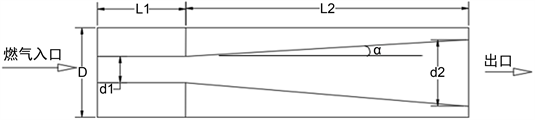Figure 2. Schematic diagram of microburnerTable 1. Structural parameters of three burners

1) 预混燃气为不可压缩理想气体，在进入燃烧器时已混合均匀；

2) 忽略气体的辐射作用；

3) 忽略气体的体积力；

4) 忽略燃烧器壁面的催化作用；

5) 忽略浓度梯度产生的粘性热耗散效应和Dufour效应；

6) 忽略入口与出口处壁面带来的环境热损失。

2.2. 微型燃烧器的数学模型

$\frac{\partial \left(\rho u\right)}{\partial x}+\frac{\partial \left(\rho v\right)}{\partial y}+\frac{\partial \left(\rho w\right)}{\partial z}=0$ (1)

$\frac{\partial \left(\rho uu\right)}{\partial x}+\frac{\partial \left(\rho uv\right)}{\partial y}+\frac{\partial \left(\rho uv\right)}{\partial z}=-\frac{\partial p}{\partial x}+\frac{\partial }{\partial x}\left(\mu \frac{\partial u}{\partial x}\right)+\frac{\partial }{\partial y}\left(\mu \frac{\partial v}{\partial y}\right)+\frac{\partial }{\partial z}\left(\mu \frac{\partial w}{\partial z}\right)$ (2)

$\frac{\partial \left(\rho vu\right)}{\partial x}+\frac{\partial \left(\rho vv\right)}{\partial y}+\frac{\partial \left(\rho wv\right)}{\partial z}=-\frac{\partial p}{\partial y}+\frac{\partial }{\partial x}\left(\mu \frac{\partial u}{\partial x}\right)+\frac{\partial }{\partial y}\left(\mu \frac{\partial v}{\partial y}\right)+\frac{\partial }{\partial z}\left(\mu \frac{\partial w}{\partial z}\right)$ (3)

$\frac{\partial }{\partial x}\left({k}_{w}\frac{\partial T}{\partial x}\right)+\frac{\partial }{\partial y}\left({k}_{w}\frac{\partial T}{\partial y}\right)+\frac{\partial }{\partial z}\left({k}_{w}\frac{\partial T}{\partial z}\right)=0$ (4)

$\begin{array}{l}\frac{\partial \left(\rho hu\right)}{\partial x}+\frac{\partial \left(\rho hv\right)}{\partial y}+\frac{\partial \left(\rho hw\right)}{\partial z}\\ =\frac{\partial }{\partial x}\left({k}_{f}\frac{\partial T}{\partial x}\right)+\frac{\partial }{\partial y}\left({k}_{f}\frac{\partial T}{\partial y}\right)+\frac{\partial }{\partial z}\left({k}_{f}\frac{\partial T}{\partial z}\right)+\sum _{i}\frac{\partial }{\partial x}\left({h}_{i}\rho {D}_{i.m}\frac{\partial {Y}_{i}}{\partial x}\right)\\ \text{\hspace{0.17em}}\text{\hspace{0.17em}}\text{ }+\sum _{i}\frac{\partial }{\partial y}\left({h}_{i}\rho {D}_{i.m}\frac{\partial {Y}_{i}}{\partial x}\right)+\sum _{i}\frac{\partial }{\partial z}\left({h}_{i}\rho {D}_{i.m}\frac{\partial {Y}_{i}}{\partial x}\right)+\sum _{i}{h}_{i}{W}_{i}\end{array}$ (5)

$\frac{\partial \left(\rho hu\right)}{\partial x}+\frac{\partial \left(\rho hv\right)}{\partial y}+\frac{\partial \left(\rho hw\right)}{\partial z}=\sum _{i}\frac{\partial }{\partial x}\left({D}_{i.m}\frac{\partial {Y}_{i}}{\partial x}\right)+\sum _{i}\frac{\partial }{\partial y}\left({D}_{i.m}\frac{\partial {Y}_{i}}{\partial x}\right)+\sum _{i}\frac{\partial }{\partial z}\left({D}_{i.m}\frac{\partial {Y}_{i}}{\partial x}\right)+{W}_{i}$ (6)

$\frac{\partial }{\partial x}\left({k}_{w}\frac{\partial T}{\partial x}\right)+\frac{\partial }{\partial y}\left({k}_{w}\frac{\partial T}{\partial y}\right)+\frac{\partial }{\partial z}\left({k}_{w}\frac{\partial T}{\partial z}\right)=0$ (7)

2.3. 反应机理Table 2.19 step reaction mechanism between hydrogen and air

$k=A{T}^{\beta }\mathrm{exp}\left(-{E}_{K}/RT\right)$ (8)

2.4. 边界条件的设置

2.5. 模型验证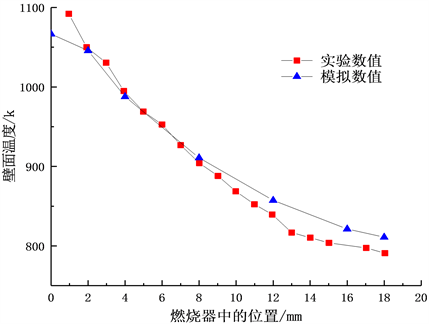Figure 3. Comparison and verification between experiment and simulation

1) 将燃烧器的流体通道的中心线上的最高燃烧温度定义为燃烧器的最高燃烧温度。

2) 将燃烧器流体通道的中心线上的OH浓度最高的位置所对应的流体速度定义为燃烧器的火焰传播速度。

3) 氢气与空气的19步反应机理中反应最慢的基元反应是第12步反应，即H2 + M = H + H + Mb，所以将燃烧器流体通道的中心线上，第12步反应最快的位置即为火焰的位置。

3. 结果与分析

3.1. 扩展角对燃烧器中的流速分布的影响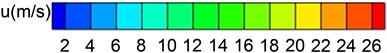(a) 扩展角为0.72˚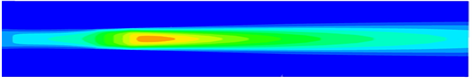(b) 扩展角为0.36˚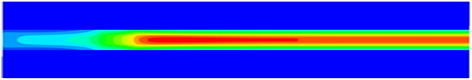(c) 扩展角为0˚

Figure 4. Cloud chart of fluid velocity distribution in burners with different spreading anglesFigure 5. Flame propagation velocities of burners with different expansion angles at different inlet velocity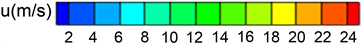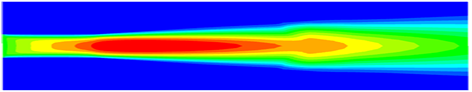(a) 入口速度为10 m/s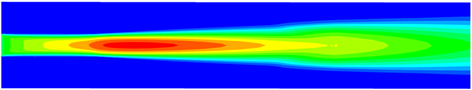(b) 入口速度为11 m/s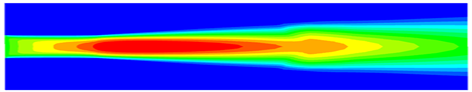(c) 入口速度为12 m/s

Figure 6. Velocity distribution cloud chart at different inlet velocities in a burner with an expansion angle of 0.72˚

3.2. 扩展角对燃烧器中的温度分布影响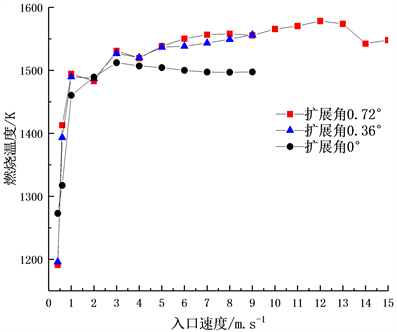Figure 7. Maximum combustion temperature at different inlet velocities in burners with different expansion angles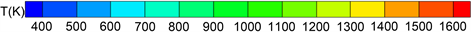(a) 扩展角为0.72˚(b) 扩展角为0.36˚(c) 扩展角为0˚

Figure 8. Cloud chart of temperature distribution in burners with different expansion angles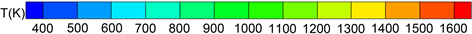(a) 入口速度为7 m/s(b) 入口速度为8 m/s(c) 入口速度为9 m/s

Figure 9. Cloud chart of temperature distribution at different inlet velocities in a burner with 0˚ expansion angle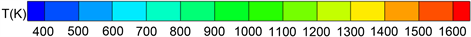(a) 入口速度为7 m/s(b) 入口速度为8 m/s(c) 入口速度为9 m/s

Figure 10. Cloud chart of temperature distribution at different inlet velocities in a burner with 0.36˚ expansion angle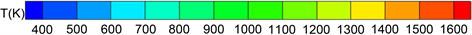(a) 入口速度为10 m/s(b) 入口速度为11 m/s(c) 入口速度为12 m/s

Figure 11. Cloud chart of temperature distribution at different inlet velocities in a burner with 0.72˚ expansion angleFigure 12. The effect of inlet velocity on fluid temperature distribution in a burner with an expansion angle of 0.72˚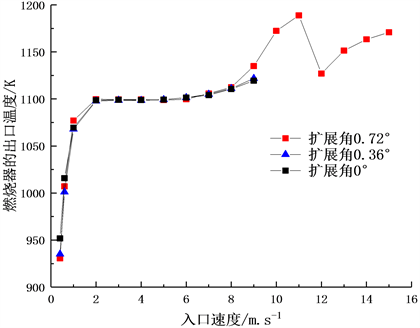Figure 13. Effect of inlet velocity of burner with different expansion angles on gas outlet temperature

3.3. 扩展角对燃烧器中燃烧产物分布的影响Figure 14. OH concentration distribution in burners with different spreading angles(a) 扩展角为0.72˚(b) 扩展角为0.36˚(c) 扩展角为0˚

Figure 15. Cloud chart of OH concentration distribution in burners with different spreading angles

3.4. 扩展角对燃烧器的火焰位置的影响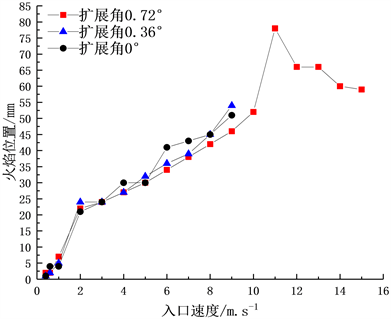Figure 16. Flame position at different inlet velocities in burners with different expansion angles

4. 结论

1) 扩展角为0.72˚的燃烧器具有更大的速度极限和更低的贫燃极限。其中氢气体积分数为10%时，扩展角为0.72˚的燃烧器的速度极限达到了0.4~15 m/s；扩展角为0.36˚和扩展角为0˚的燃烧器的速度极限为0.4~9 m/s。

2) 扩展角为0˚的燃烧器在入口速度较高时表现出了更低的燃烧温度，更高的火焰传播速度；扩展角为0.72˚的燃烧器在入口速度为11 m/s时火焰中心最靠近出口位置，这对燃烧器的燃烧不利。

3) 三种燃烧器在共有的速度极限范围内不同入口速度下的出口温度相同，不受燃烧器结构的影响。

Simulation Study on the Influence of Micro Burner Expansion Angle on Hydrogen/Air Premixed Combustion Characteristics[J]. 建模与仿真, 2021, 10(01): 93-106. https://doi.org/10.12677/MOS.2021.101010

1. 1. 张冬至, 胡国清. 微机电系统关键技术及其研究进展[J]. 压电与声光, 2010, 32(3): 513-520. http://dx.chinadoi.cn/10.3969/j.issn.1004-2474.2010.03.051

2. 2. 邵霞, 潘剑锋, 唐爱坤, 胡松, 侯智勇. 微尺度催化燃烧表面反应对气相反应的影响[J]. 农业机械学报, 2015, 46(12): 391-396. http://dx.chinadoi.cn/10.6041/j.issn.1000-1298.2015.12.053

3. 3. 刘子琨. 微尺度平板燃烧器中甲烷、二甲醚催化燃烧研究[D]: [硕士学位论文]. 杭州: 浙江大学, 2017.

4. 4. Chen, G.B., Chen, C.P., Wu, C.Y. and Chao, Y.-C. (2007) Effects of Catalytic Walls on Hydrogen/Air Combustion Inside a Micro-Tube. Applied Catalysis A: General, 332, 89-97. https://doi.org/10.1016/j.apcata.2007.08.011

5. 5. Zhong, B.J. and Wang, J.H. (2010) Experimental Study on Premixed CH4/Air Mixture Combustion in Micro Swiss-Roll Combustors. Combustion and Flame, 157, 2222-2229. https://doi.org/10.1016/j.combustflame.2010.07.014

6. 6. 李军伟, 钟北京, 王宁飞, 魏志军. 空气槽对微型双通道螺旋型过量焓燃烧器工作特性的影响[J]. 中国电机工程学报, 2010, 30(2): 40-45.

7. 7. 刘伟, 陈琪. Swiss-Roll型微燃烧器的燃烧数值模拟[J]. 工业加热, 2013, 42(3): 27-28, 39. http://dx.chinadoi.cn/10.3969/j.issn.1002-1639.2013.03.009

8. 8. Yang, W.M., Chou, S.K., Shu, C., Li, Z.W. and Xue, H. (2002) Combustion in Micro-Cylindrical Combustors with and without a Backward Facing Step. Applied Thermal Engineering, 22, 1777-1787. https://doi.org/10.1016/S1359-4311(02)00113-8

9. 9. Yang, W.M., Chou, S.K., Shu, C., Li, Z.W. and Xue, H. (2005) Study of Catalytic Combustion and Its Effect on Microthermophotovoltaic Power Generators. Journal of Physics D: Applied Physics, 38, 4252. https://doi.org/10.1088/0022-3727/38/23/018

10. 10. Yang, W.M., Chou, S.K., Shu, C., Li, Z.W., Xue, H., Li, D.T., et al. (2003) Microscale Combustion Research for Application to Micro Thermophotovoltaic systems. Energy Conver-sion and Management, 44, 2625-2634. https://doi.org/10.1016/S0196-8904(03)00024-4

11. 11. 邓尘, 杨卫娟, 王威, 周俊虎, 刘建忠, 岑可法. 微型缩放喷管中氢气/空气预混燃烧实验研究[J]. 中国电机工程学报, 2013, 34(2): 260-265. http://dx.chinadoi.cn/10.13334/j.0258-8013.pcsee.2014.02.007

12. 12. Giovangigli, V. and Smooke, M.D. (1987) Extinction of Strained Premixed Laminar Flames with Complex Chemistry. Combustion Science and Technology, 53, 23-49. https://doi.org/10.1080/00102208708947017

13. 13. Tang, A.K., Xu, Y.M., Shan, C.X., Pan, J.F. and Liu, Y.X. (2015) A Comparative Study on Combustion Characteristics of Methane, Propane and Hydrogen Fuels in a Mi-cro-Combustor. International Journal of Hydrogen Energy, 40, 16587-16596. https://doi.org/10.1016/j.ijhydene.2015.09.101

14. 14. Norton, D.G. and Vlachos, D.G. (2003) Combustion Char-acteristics and Flame Stability at the Microscale: A CFD Study of Premixed Methane/Air Mixtures. Chemical Engi-neering Science, 58, 4871-4882. https://doi.org/10.1016/j.ces.2002.12.005

15. 15. Norton, D.G. and Vlachos, D.G. (2004) A CFD Study of Pro-pane/Air Microflame Stability. Combustion and Flame, 138, 97-107. https://doi.org/10.1016/j.combustflame.2004.04.004

16. NOTES

*通讯作者。